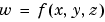MATLAB Function Referencegriddata3

Data gridding and hypersurface fitting for 3-D data

Syntax

• ```w = griddata3(x,y,z,v,xi,yi,zi)
w = griddata3(`x,y,z,v,xi,yi,zi`,method)
```w = griddata3(x,y,z,v,xi,yi,zi,method,options)
``````

Description

```w = griddata3(x, y, z, v, xi, yi, zi) ``` fits a hypersurface of the formto the data in the (usually) nonuniformly spaced vectors (`x`, `y`, `z`, `v`). `griddata3` interpolates this hypersurface at the points specified by (`xi`,`yi`,`zi`) to produce `w`. `w` is the same size as `xi`, `yi`, and `zi`.

(`xi`,`yi`,`zi`) is usually a uniform grid (as produced by `meshgrid`) and is where `griddata3` gets its name.

```w = griddata3(x,y,z,v,xi,yi,zi,method) ``` defines the type of surface that is fit to the data, where `method` is either:

 `'linear'` Tesselation-based linear interpolation (default) `'nearest'` Nearest neighbor interpolation

If `method` is `[]`, the default `'linear'` method is used.

`w = griddata3(x,y,z,v,xi,yi,zi,method,options)` specifies a cell array of strings `options` to be used in Qhull via `delaunayn`.

If `options` is `[]`, the default options are used. If `options` is `{''}`, no options are used, not even the default.

Algorithm

The `griddata3` methods are based on a Delaunay triangulation of the data that uses Qhull . For information about Qhull, see http://www.qhull.org/. For copyright information, see http://www.qhull.org/COPYING.html.

See Also

`delaunayn`, `griddata`, `griddatan`, `meshgrid`

Reference

  Barber, C. B., D.P. Dobkin, and H.T. Huhdanpaa, "The Quickhull Algorithm for Convex Hulls," ACM Transactions on Mathematical Software, Vol. 22, No. 4, Dec. 1996, p. 469-483. Available in PDF format at http://www.acm.org/pubs/citations/journals/toms/1996-22-4/p469-barber.

  National Science and Technology Research Center for Computation and Visualization of Geometric Structures (The Geometry Center), University of Minnesota. 1993.

© 1994-2005 The MathWorks, Inc.